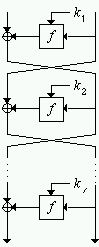### Question 56. What is a Feistel Cipher?Figure 2. Feistel Cipher

Feistel ciphers [Fei73] are a special class of iterated block ciphers (see Question 55) where the ciphertext is calculated from the plaintext by repeated application of the same transformation or round function. Feistel ciphers are also sometimes called DES-like ciphers (see Question 64).

In a Feistel cipher (see Figure 2), the text being encrypted is split into two halves. The round function f is applied to one half using a subkey and the output of f is exclusive-ored with the other half. The two halves are then swapped. Each round follows the same pattern except for the last round where there is no swap.

A nice feature of a Feistel cipher is that encryption and decryption are structurally identical, though the subkeys used during encryption at each round are taken in reverse order during decryption.

It is possible to design iterative ciphers that are not Feistel ciphers, yet whose encryption and decryption (after a certain re-ordering or re-calculation of variables) are structurally the same. One such example is IDEA (see Question 77).